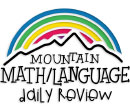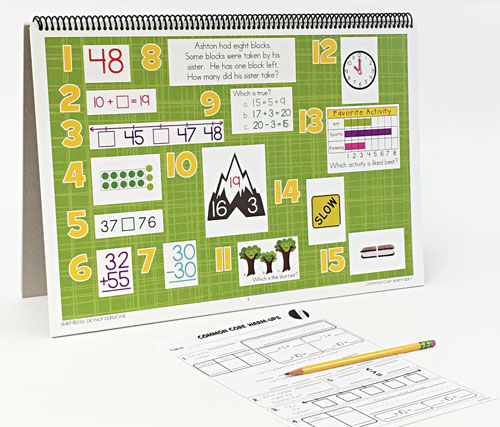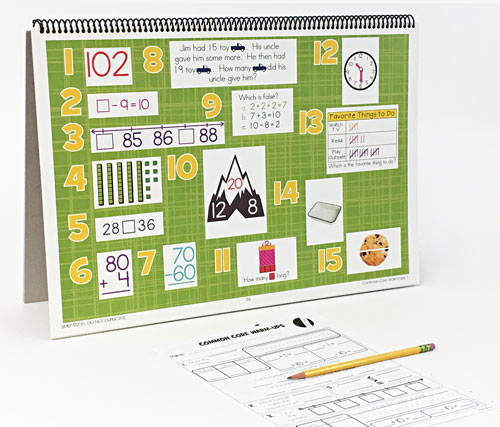WHAT YOU GET:
• 32 WEEKS OF DAILY REVIEW
• INSTRUCTIONS
• ONE MASTER STUDENT RESPONSE SHEET
• HEAVY DUTY STAND
• KNOWLEDGEABLE STAFF FOR ANY ASSISTANCE

This 1st GRADE COMMON CORE DAILY REVIEW MATH CENTER EDITION is the DAILY REVIEW your students need to MASTER the concepts you have taught.

Research has proven that LONG-TERM MASTERY comes from SPIRAL REVIEW, also called DISTRIBUTED PRACTICE AND SPACED REPETITION.

The CENTER EDITION is designed for small group review, such as rotations, scoots, centers, or math-around-the-room. It is a smaller version (13"x19") of our Bulletin Board Kit. It can easily be set up and taken down as needed.
1. 1.NBT.A.1 Count to 120, starting at any number less than 120. In this range, read and write numerals and represent a number of objects with a written numeral.
1.NBT.B.2.A 10 can be thought of as a bundle of ten ones — called a "ten."
1.NBT.B.2.B The numbers from 11 to 19 are composed of a ten and one, two, three, four, five, six, seven, eight, or nine ones.
1.NBT.B.2.C The numbers 10, 20, 30, 40, 50, 60, 70, 80, 90 refer to one, two, three, four, five, six, seven, eight, or nine tens (and 0 ones).
2. 1.OA.B.3 Apply properties of operations as strategies to add and subtract.
1.OA.B.4 Understand subtraction as an unknown-addend problem.
1.OA.C.5 Relate counting to addition and subtraction.
1.OA.D.8 Determine the unknown whole number in an addition or subtraction equation relating three whole numbers.
3. 1.NBT.B.2.A 10 can be thought of as a bundle of ten ones — called a "ten."
1.NBT.B.2.B The numbers from 11 to 19 are composed of a ten and one, two, three, four, five, six, seven, eight, or nine ones.
1.NBT.B.2.C The numbers 10, 20, 30, 40, 50, 60, 70, 80, 90 refer to one, two, three, four, five, six, seven, eight, or nine tens (and 0 ones).
1.NBT.C.5 Given a two-digit number, mentally find 10 more or 10 less than the number, without having to count; explain the reasoning used.
4. 1.NBT.A.1 Count to 120, starting at any number less than 120. In this range, read and write numerals and represent a number of objects with a written numeral.
5. 1.NBT.B.3 Compare two two-digit numbers based on meanings of the tens and ones digits, recording the results of comparisons with the symbols >, =, and <.
6. 1.NBT.C.4 Add within 100, including adding a two-digit number and a one-digit number, and adding a two-digit number and a multiple of 10, using concrete models or drawings and strategies based on place value, properties of operations, and/or the relationship between addition and subtraction; relate the strategy to a written method and explain the reasoning used. Understand that in adding two-digit numbers, one adds tens and tens, ones and ones; and sometimes it is necessary to compose a ten.
7. 1.NBT.C.6 Subtract multiples of 10 in the range 10-90 from multiples of 10 in the range 10-90 (positive or zero differences), using concrete models or drawings and strategies based on place value, properties of operations, and/or the relationship between addition and subtraction; relate the strategy to a written method and explain the reasoning used.
8. 1.OA.A.1 Use addition and subtraction within 20 to solve word problems involving situations of adding to, taking from, putting together, taking apart, and comparing, with unknowns in all positions, e.g., by using objects, drawings, and equations with a symbol for the unknown number to represent the problem.
1.OA.A.2 Solve word problems that call for addition of three whole numbers whose sum is less than or equal to 20, e.g., by using objects, drawings, and equations with a symbol for the unknown number to represent the problem.
1.OA.C.6 Add and subtract within 20, demonstrating fluency for addition and subtraction within 10.
1.OA.C.8 Determine the unknown whole number in an addition or subtraction equation relating three whole numbers.
9. 1.OA.D.7 Understand the meaning of the equal sign, and determine if equations involving addition and subtraction are true or false.
10. 1.OA.B.3 Apply properties of operations as strategies to add and subtract.
1.OA.B.4 Understand subtraction as an unknown-addend problem.
1.OA.C.6 Add and subtract within 20, demonstrating fluency for addition and subtraction within 10.
11. 1.MD.A.1 Order three objects by length; compare the lengths of two objects indirectly by using a third object.
1.MD.A.2 Express the length of an object as a whole number of length units, by laying multiple copies of a shorter object (the length unit) end to end; understand that the length measurement of an object is the number of same-size length units that span it with no gaps or overlaps.
12. 1.MD.B.3 Tell and write time in hours and half-hours using analog and digital clocks.
13. 1.MD.C.4 Organize, represent, and interpret data with up to three categories; ask and answer questions about the total number of data points, how many in each category, and how many more or less are in one category than in another.
14. 1.G.A.1 Distinguish between defining attributes (e.g., triangles are closed and three-sided) versus non-defining attributes (e.g., color, orientation, overall size); build and draw shapes to possess defining attributes.
15. 1.G.A.3 Partition circles and rectangles into two and four equal shares, describe the shares using the words halves, fourths, and quarters, and use the phrases half of, fourth of, and quarter of. Describe the whole as two of, or four of the shares. Understand for these examples that decomposing into more equal shares creates smaller shares.
ONLINE REVIEW VS.
CENTER EDITION VS.
BULLETIN BOARD KIT
ONLINE REVIEW
Is projected onto an interactive white board, creating a virtual board. It can be viewed by the entire classroom. Answers can be displayed onto the board at the click of a button. Up to seven questions can be isolated and magnified.

CENTER EDITION
Is organized on a 13"x19" flip chart. It can be viewed by 5-6 students at a time. It was designed for small group review, such as rotations, scoots, centers, or math-around-the-classroom. It has an answer key, heavy-duty stand, and high-gauged coil.

BULLETIN BOARD KIT
It is designed for total classroom view. It hangs on bulletin boards, walls, white boards, pocket charts, and science display boards. Once hung, it can remain displayed for the entire year. The cards can be interchanged, creating flexible and adjustable DAILY REVIEW.
\$95.95 QTY:
Centers   |   1st Grade Common Core Math